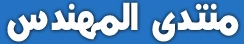# UTILIZATION OF DYNAMIC RELAXATION METHOD IN SOLVING ORDINARY AND PARTIAL DIFFERENTIAL EQUATIONS OF RECTANGULAR ENGINEERING STRUCTURES

The method of dynamic relaxation in its early stages of development was perceived as a numerical finite difference technique. It was first used to analyze structures, then skeletal and cable structures, and plates. The method relies on a discretized continuum in which the mass of the structure is assumed to be concentrated at given points (i.e. nodes) on the surface. The system of concentrated masses oscillates about the equilibrium position under the influence of out of balance forces. With time, it comes to rest under the influence of damping. The iterative scheme reflects a process, in which static equilibrium of the system is achieved by simulating a pseudo dynamic process in time. In its original form, the method makes use of inertia term, damping term and time increment. The basics of this research paper stand on the ordinary and partial differential equations, which value the price of an option by using dynamic relaxation (DR) techniques. The study of partial differential equations in complete generality is a vast undertaking. As almost all of them are not possible to solve analytically we must rely on numerical methods, and the most popular ones are the finite differences methods coupled with dynamic relaxation techniques. With this research paper i do not intend to become an expert in few hours in order to solve differential equations numerically, but develop both intuition and technical strength required to survive when such a problem needs to be solved.UTILIZATION OF DYNAMIC RELAXATION METHOD IN SOLVING ORDINARY AND PARTIAL DIFFERENTIAL EQUATIONS.pdf (559.8% u)

1 Like